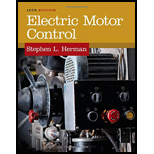# Explain the reason why direct current (DC) controller can be called as a counter emf controller.### Electric Motor Control

10th Edition
Herman
Publisher: CENGAGE L
ISBN: 9781133702818### Electric Motor Control

10th Edition
Herman
Publisher: CENGAGE L
ISBN: 9781133702818

#### Solutions

Chapter 46, Problem 1SQ
To determine

## Explain the reason why direct current (DC) controller can be called as a counter emf controller.

Expert Solution

DC controller can be called as a counter emf controller because the counter emf of the armature is used for controlling the motor acceleration.

### Explanation of Solution

In DC motor, the generated counter electromotive force is proportional to the speed of the motor for constant field. The counter emf can differ from the applied voltage only by armature drop.

DC controller can automatically adjust the starting time intervals, based upon the connected load. If heavy load is connected, it has more inertia to overcome; therefore, long time is required to generate the counter emf. When the DC motor starts, counter emf produced across the armature is very low. As the acceleration of motor increases, the counter emf increases. If the voltage value across the armature reaches a particular value, the starting resistance can be reduced at the correct time by actuating the relay. Therefore, the counter emf is mainly responsible to control the acceleration of motor.

Conclusion:

Thus, the DC controller can be called as a counter emf controller because the counter emf of the armature is used for controlling the motor acceleration.

### Want to see more full solutions like this?

Subscribe now to access step-by-step solutions to millions of textbook problems written by subject matter experts!

### Want to see more full solutions like this?

Subscribe now to access step-by-step solutions to millions of textbook problems written by subject matter experts!